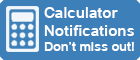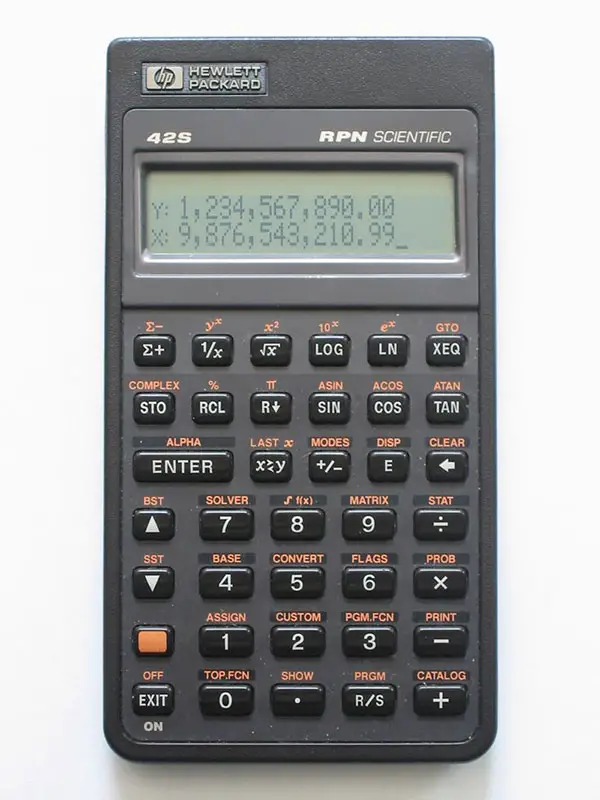Calculator Library
Free Online CalculatorsHome » 87 Calculators » Blog » What Does RPN Mean On A Calculator?

Huge Domain Name Sale | Free Domains
Domain names on sale at crazy prices. Register a new domain for FREE when you buy another service.

## WHAT DOES RPN MEAN ON A CALCULATOR?"RPN" stands for Reverse Polish Notation and is also known as Postfix Notation. It's a mathematical notation where the operators are entered after the numbers, and this results in the most obvious difference for an RPN calculator — there is no need for an equals button.

This contrasts to Polish notation (PN), where the operators are entered before the numbers. "Polish" in the name refers to the inventor of this notation, Polish logician Jan Łukasiewicz.

As an example, if you're performing the calculation of 1 + 2 = 3 on a standard or algebraic notation calculator, the button sequence would be 1 + 2 = resulting in the answer "3" displayed on the screen. However, the same calculation on an RPN calculator would require the button sequence 1 Enter 2 + to see the answer "3".

This process also works for unlimited multiple numbers in sequence. For example, you can add 1 Enter 2 + 3 + 4 + to see the answer "10" or multiply 1 Enter 2 x 3 x 4 x to see the answer "24".

Another RPN example involves single-number calculations. Here, the number is still entered first, followed by the operation key to see the result. For example, to calculate e3 the button sequence would be 3 ex.

9 Enter 2 x
8 Enter 3 x +
7 Enter 4 x -
For many users, a big advantage of RPN is that the calculation process is similar to how you'd calculate on paper. This can be illustrated with a more complicated example involving chain calculations. Here is the button sequence to calculate (9 × 2) + (8 × 3) – (7 × 4):

When using RPN, there's no need for parentheses or brackets in calculations. RPN is also popular because complex calculations can be simplified by reducing keystrokes leading to quicker and easier problem-solving. In more extended calculations, it also lets you see interim results, not just the final answer. This allows you to check for errors at each step and can also be beneficial in teaching situations to show students how a result is calculated.

It can be helpful to think of RPN in terms of a stack structure with several levels, commonly 3 or 4. To do this, take a mathematical expression and read it from left to right. If a number is entered, this value is pushed onto the stack. If an operator is entered, this processes two values from the top of the stack and pushes the result of the operation back onto the stack.

Despite the advantages of RPN, many people prefer to use a standard or algebraic mode for some calculations. As a result, some calculators allow you to switch between RPN mode and algebraic mode to satisfy this preference.

RPN was relatively unknown before the 1970s when Hewlett-Packard began using it in all of their calculators and still uses it in some models today. The famous Hewlett-Packard HP-12C Financial Programmable Calculator is amongst those calculators using RPN. However, more recently, RPN has decreased in popularity since algebraic notation calculators such as the TI-84 have become the standard in many schools and universities.

As a final point, it's important to note that RPN can also be found in computer science, where it's used in stack-based programming languages, including PostScript and Forth. However, calculators are the focus of this article so we've limited our discussion to that subject.

 Previous Post« What Does E Mean On A Calculator? Next PostHow To Change A Calculator To Degrees? »